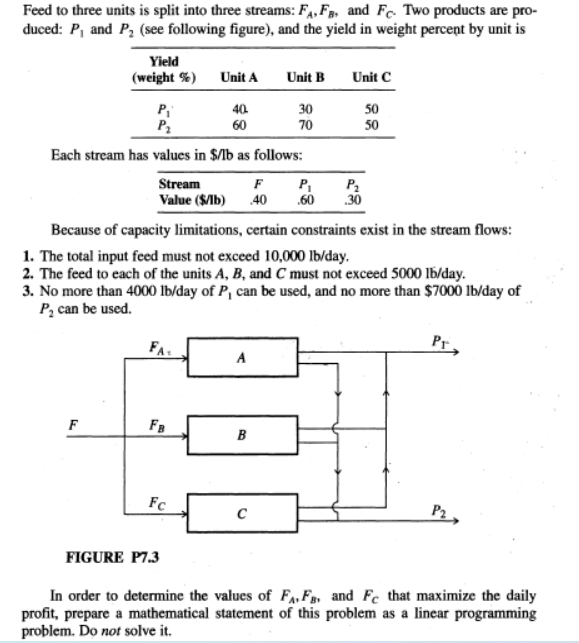# Question Feed to three units is split into three streams: F4,FB, and Fc. Two products are pro- duced: P, and P2 (see following figure), and the yield in weight percent by unit is Yield (weight %) Unit A Unit B Unit C P 40 30 50 P2 60 70 50 Each stream has values in \$/lb as follows: Stream F P, P2 Value (SIL) .40 .60 .30 Because of capacity limitations, certain constraints exist in the stream flows: 1. The total input feed must not exceed 10,000 lb/day. 2. The feed to each of the units A, B, and C must not exceed 5000 lb/day. 3. No more than 4000 lb/day of P, can be used, and no more than \$7000 lb/day of P, can be used. Pr, А F FB B Fc с P2 FIGURE P7.3 In order to determine the values of FFs, and Fc that maximize the daily profit, prepare a mathematical statement of this problem as a linear programming problem. Do not solve it.WG2ZN6 The Asker · Electrical EngineeringTranscribed Image Text: Feed to three units is split into three streams: F4,FB, and Fc. Two products are pro- duced: P, and P2 (see following figure), and the yield in weight percent by unit is Yield (weight %) Unit A Unit B Unit C P 40 30 50 P2 60 70 50 Each stream has values in \$/lb as follows: Stream F P, P2 Value (SIL) .40 .60 .30 Because of capacity limitations, certain constraints exist in the stream flows: 1. The total input feed must not exceed 10,000 lb/day. 2. The feed to each of the units A, B, and C must not exceed 5000 lb/day. 3. No more than 4000 lb/day of P, can be used, and no more than \$7000 lb/day of P, can be used. Pr, А F FB B Fc с P2 FIGURE P7.3 In order to determine the values of FFs, and Fc that maximize the daily profit, prepare a mathematical statement of this problem as a linear programming problem. Do not solve it.
More
Transcribed Image Text: Feed to three units is split into three streams: F4,FB, and Fc. Two products are pro- duced: P, and P2 (see following figure), and the yield in weight percent by unit is Yield (weight %) Unit A Unit B Unit C P 40 30 50 P2 60 70 50 Each stream has values in \$/lb as follows: Stream F P, P2 Value (SIL) .40 .60 .30 Because of capacity limitations, certain constraints exist in the stream flows: 1. The total input feed must not exceed 10,000 lb/day. 2. The feed to each of the units A, B, and C must not exceed 5000 lb/day. 3. No more than 4000 lb/day of P, can be used, and no more than \$7000 lb/day of P, can be used. Pr, А F FB B Fc с P2 FIGURE P7.3 In order to determine the values of FFs, and Fc that maximize the daily profit, prepare a mathematical statement of this problem as a linear programming problem. Do not solve it.